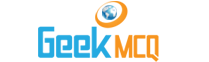# Engineering :: Hydraulics and Fluid Mechanics

1.  The mass per unit volume of a liquid at a standard temperature and pressure is called
 A. specific weight B. mass density C. specific gravity D. none of these

2.  The volume per unit mass of a liquid is called specific volume.
 A. Yes B. No

3.  The weight per unit volume of a liquid at a standard temperature and pressure is called
 A. specific weight B. mass density C. specific gravity D. none of these

4.  The specific weight of water in S.I. units is taken as
 A. 9.81 kN/m3 B. 9.81 x 103 N/m3 C. 9.81 x 10-6 N/mm3 D. any of these

5.  The ratio of specific weight of a liquid to the specific weight of pure water at a standard temperature is called
 A. density of liquid B. specific gravity of liquid C. compressibility of liquid D. surface tension of liquid

6.  The specific gravity has no units.
 A. Agree B. Disagree

7.  The specific gravity of water is taken as
 A. 0.001 B. 0.01 C. 0.1 D. 1

8.  The specific weight of sea water is
 A. same as B. less than C. more than

9.  The density of a liquid in kg / m3 is numerically equal to its specific gravity.
 A. True B. False

10.  The specific weight is also known as weight density.
 A. Correct B. Incorrect